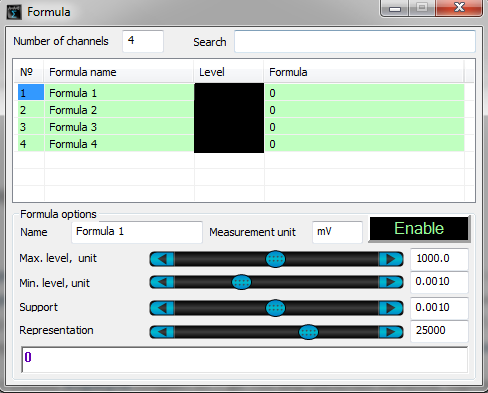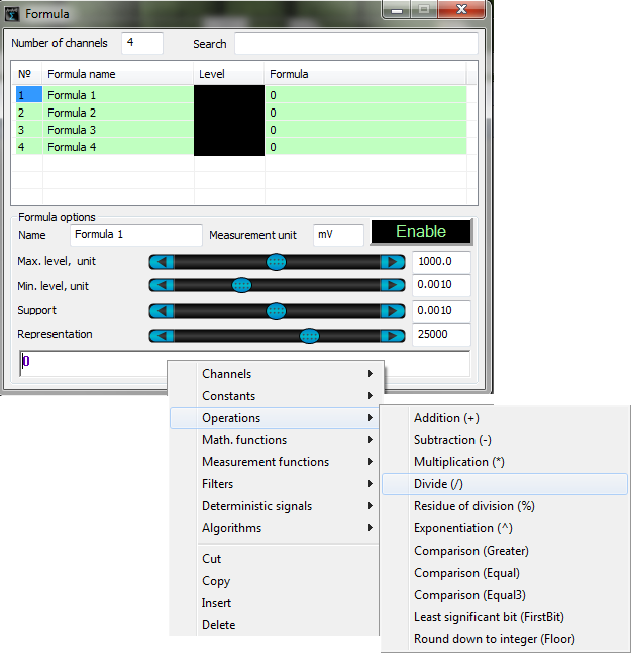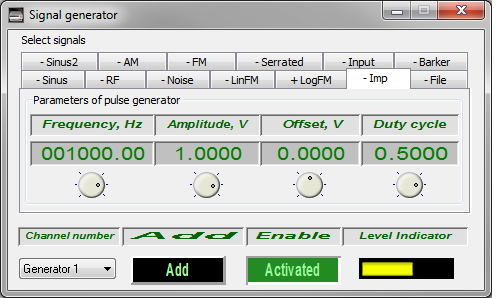# ZETLAB Formula

ZETLAB Formula is a program used for mathematical operations with signals, signal filtration, and parameters measurements, producing signals with various shapes. ZETLAB Formula is a virtual measuring tool allowing to perform arithmetical and logical operations and filtration of constant data flows received from ADC and DAC modules and virtual channels in real-time mode. In the post-processing mode, it is possible to perform various operations with the files created by means of “Signals recording” program.

ZETLAB Formula allows to create from 1 up to 100 virtual channels. It is also possible to use several Formula programs simultaneously.## ZETLAB Formula: parameters

Each signal created by Formula program is sent to ZETLAB server, thus, it becomes available for further processing with any program from the ZETLAB scope. Each channel of Formula has a name specified in “Name of the channel” section.

Section “Measuring unit” specifies the unit of measurements for a particular channel. For instance, if Formula program is used for the calculation of secondary parameters of the stress-strain state based on the signals received from the strain-gauge sensors, the signal will be displayed in measurement units of the calculated parameters.

The sections “Max. level, unit” and “Min. level, unit” specify the range of the signal in corresponding measuring units used for calculation of the relative level specified in the field “Integral level“. The set values do not affect the level of the created signals since the calculation is performed in accordance with the set formula.

Section “Support” contains a value for calculation of signal level in dB, which is used by such programs as DC voltmeter, Narrow-band spectrum, and other programs, that depict measurements results in dB.

Representation frequency” section is used to set signal sampling frequency (i.e. the number of signal points per second).

The bottom part of the program window contains a field with the formula used for signal calculation. You can enter the formula using the keyboard or a context menu (to enable the context menu, right-click the area).The context menu for entering the formula contains the following elements:

• #### Channels

list of ZET-server channels

• #### Constants

list of constants

• #### Operations

list of mathematical operations

• #### Math. functions

list of mathematical functions

• #### Meas. functions

list of measurement functions

• #### Filters

list of filters

• #### Deterministic signals

list of deterministic signals

• #### Algorithms

list of available algorithms

Standard commands like “Cut“, “Copy“, “Paste” and “Delete” are also available for various operations with formula components.

## Changing signal frequency and phase

using ZETLAB Formula

Let us consider that we have a sinusoidal signal which has been previously measured, and now we have to produce a signal of the same amplitude with a different phase and frequency using DAC.

We are going to use  ZET 210 with 16 ADC output channels and 2 DAC channels.  We will use the first channel of DAC for generation of sine signal with sweeping frequency using the program “Signal generator”.Let us connect the output of the first DAC channel to the input of the first ADC channel. Thus, we will have a signal for further processing.Let us enable measurement of input signal frequency by starting “Frequency meter”. It allows to create a virtual channel “Frequency Sig_1_1” with the measurements results of signal frequency.Start the “Formula” program, create “Signal” channel with a sine signal having the same amplitude, but a different frequency. The frequency will be changed based on multiplication law.Create a second channel “Signal 2” containing the sinusoidal signal from the first channel with a 45° phase shift.The signal obtained can be reproduced by the second channel of the ADC device.Delivery terms: CIP, CPT or DAP. Customs clearance and delivery is carried out by the "Cordely" custom company (included in the price of the goods). 1992 - 2023 ZETLAB Company